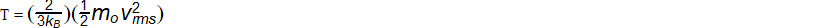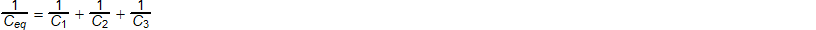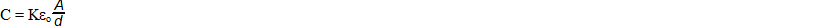### AP Physics 1 Week 6 (July 16 - July 22, 2017)

Part 1:  Algebra (Rearrange)

1.  solve for kB2. solve for vrms3.  solve for C34.  solve for Ceq5.  solve for dPart 2:  Algebra (Inverse and Direct Relationships)

6 A gas whose volume is 500 cubic centimeters is under a pressure of 30 inches of mercury at an absolute temperature of 360 Kelvin.  What pressure would be exerted by the gas if the volume rose to 570 cubic centimeters and its absolute temperature dropped to 285 Kelvin?  The pressure on any gas varies directly with its absolute temperature and inversely with its volume.  (DBW, p317, #10)

7.  The weight of an object is inversely proportional to the square of its distance from the center of the earth.  If a man weighs 147 pounds on the earths surface, what will he weigh 200 miles above the earth?  Assume the radius of the earth to be 4000 miles.

(DBW, p317, #11)

8.  The amount of bending of a beam supported at each end and centrally loaded varies jointly as the load in pounds and the cube of the length in feet and varies inversely as the cube of its depth in inches.  A beam of 12 feet long, 6 inches thick, carrying a load of 2000 pounds, bends .25 inches downward.  What is the maximum load a 16 foot beam, of the same width and material, whose depth is 4 inches can carry at the center so as to bend not more than 1.125 inches at the center?  (DBW, p317, #12)

9.   A parachute has a surface area of 150 square feet.  The resistance of the air varies as the square of the velocity and is equal to 2 pounds per square foot of opposing surface for a velocity of 30 feet per second.  If a man and a parachute together weigh 192 pounds, how fast will the man be falling when the upward pull of the parachute is equal to the downward pull of gravity?  (Camp, p187, # 7)

10.  The time, t, required to empty a tank varies inversely as the rate, r, of pumping. If a pump can empty a tank in 2.5 hours at a rate of 400 gallons per minute, how long will it take to empty a tank at 500 gallons per minute?

Part 3: Geometry/Trigonometry
(For Physics, make sure your calculator is set in degrees)

Right Triangle Review:
Pythagorean Theorem (math version) c2 = a2 + b2, (physics version) r2 = x2 + y2
r = hypotenuse of the right triangle
x = horizontal or (east-west) component of the triangle
y = vertical or (north-south) component of the triangle
q = the reference angle always between the x axis and the hypotenuse

horizontal component x = r*cos(q)  vertical component y = r*sin(q)

Draw a picture and solve each problem.
11.  When the sun is 20o above the horizon, how long is the shadow cast by a building 150 ft high?

12.  A tree 100 ft tall casts a shadow 120 ft long.  Find the angle of elevation above the horizon of the sun.

13. 14.  A ladder leans against the side of a building with its base 12 ft from the building.  How far from the ground is the top of the ladder leaning on the side of the building and how long is the ladder if it makes and angle of 70o with the ground?

15.  From the top of a lighthouse, 120 ft above the sea, the angle of depression toward a bot on the water is 15o.  How far is the boat from the base of the lighthouse?

Part 4:  Word Problems

16. Two railroad workers are together in a 0.6 mile mountain tunnel.  One walks east

and the other walks west in order to be out of the tunnel before the "express"

train comes through at 30 mph.  Each man reaches his respective end of the

tunnel in 3 minutes.  If the man walking east reaches the east entrance just as

the train enters, and the train passes the other man 0.12 miles beyond the

opening of the west end of the tunnel, at what speed did each man walk?

(DBW, p104, #29)

17. Nancy and William start at the same time from a mailbox.  Nancy travels north

while William travels west.  Williams speed is 4 mph greater than twice that of

Nancy's.  After one hour, they are 26 miles apart.  What is the speed of each

person? (DBW, p138, #18)

18. After making a trip of 126 miles, a lady found that if she had increased her average speed by 8 mph, she could have made the trip in 1 hour less time.  Find her

original speed. (DBW, p181, #4)

19. A rowing crew times itself on a 3 mile course.  The 3 miles upstream take 20

minutes, and the 3 miles downstream takes 12 minutes.  What is the speed of

the rowing crew in still water? (DBW, p182, #13)

20. With the wind behind him, a skater crossed a frozen river 1 mile wide in 3 minutes.              He skated straight into the wind on his return trip, which took 15 minutes.  Find

the speed of the wind. (DBW, p182, #14)